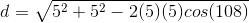# GMAT Math : DSQ: Calculating the length of a diagonal of a polygon

## Example Questions

### Example Question #6 : Polygons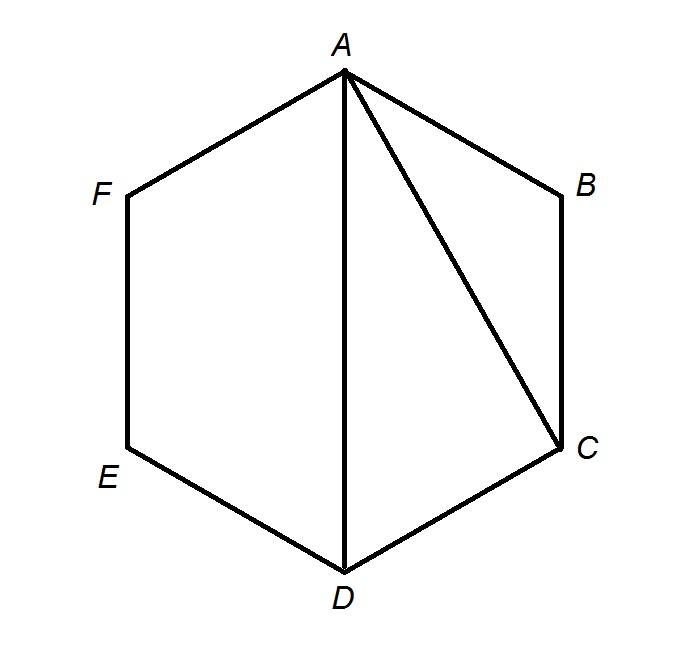The hexagon in the above diagram is regular. If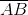has length 10, which of the following expressions is equal to the length of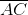?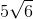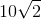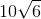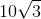Explanation:

The answer can be seen more easily by constructing the altitude of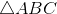from, as seen below: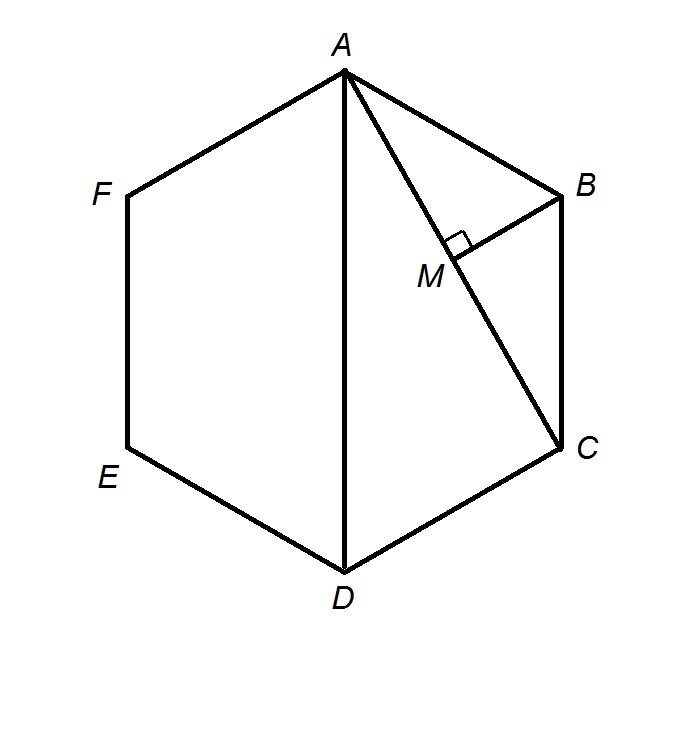Each interior angle of a hexagon measures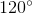,and the altitude also bisects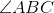, the vertex angle of isosceles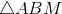is easily proved to be a 30-60-90 triangle, so by the 30-60-90 Triangle Theorem,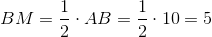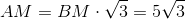The altitude also bisectsat, so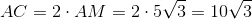.

### Example Question #7 : Polygons

Calculate the diagonal of a rectangle.

Statement 1:  The perimeter is 10.

Statement 2:  The area is 4.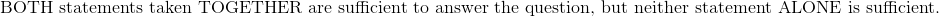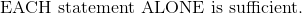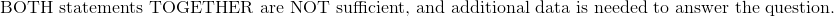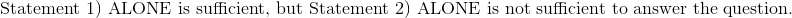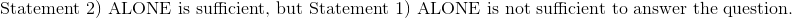Explanation:

Statement 1:  The perimeter is 10.

Given the perimeter of a rectangle is 10, set up the equation such that the sum of twice of length and width are equal to 10.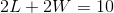There are multiple combinations of length and width that will work in this scenario.

Statement 2:  The area is 4.

Write the formula for area of a rectangle and substitute the area.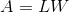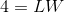Statements 1 and 2 require each other in order to solve for the length and width since there are two unknown variables.

Afterward, the Pythagorean Theorem can be used to solve for the diagonal of the rectangle.

Therefore:### Example Question #8 : Polygons

A regular pentagon has been drawn on the side of a building by some mathematically minded graffiti artists. What is the length of a diagonal across it?

1) The length of a side is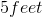.

2) Each of the internal angles is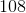degrees.

Neither of the statements, separate or together, is sufficient.

Together the two statements are sufficient.

Statement 2 alone is sufficient.

Statement 1 alone is sufficient.

Either of the statements is sufficient.

Statement 1 alone is sufficient.

Explanation:

If the pentagon is regular, then it is known that each of the internal angles isdegrees, since the sum of the five interior angles of a pentagon is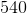degrees. Statement 2 does not give new information, nor does it give enough.

Statement 1 does, however. The length of the diagonal can be found by using the law of cosines: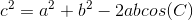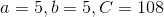and c is the length of the diagonal.